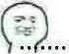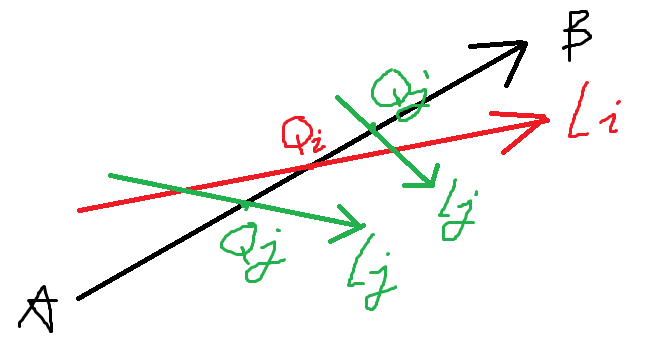# 【杂文】SCOI2020 游记

### 时间：2020-6-16

$$3$$ 道题我 $$tm$$ 考个 $$chui\ zi$$ 啊，要是有一点失误就再没有机会翻盘了。

$$rp$$

### 时间：2020-6-22

$$\text{T1}$$ 是个决策单调性优化简单 $$\text{dp}$$ 的板子。$$\text{T2}$$ 毒瘤图论，只写了 $$20pts$$ 的蒟蒻二分图。$$\text{T3}$$ 分别写个 暴力树剖 $$\text{LCA}$$$$n\leqslant 5000$$、点分树上维护 $$\text{vector}$$ 解决无修改、点分树上维护 $$\text{vector}$$ + $$\text{CDQ}$$ 套线段树解决离线、树状数组套主席树解决链，总共能获得 $$80pts$$ 的部分分。### 时间：2020-6-23

$$\text{T1}$$ $$66pts$$ 的暴力 $$\text{DP}$$ 被卡常到 $$39pts$$$$\text{T2}$$ 最后半小时想出了正解但没时间写了（$$\text{Splay}$$ 维护 $$\text{DP}$$）。

### 时间：2020-6-27 (Day1)

#### 下午

$$\text{T1}$$ 一眼 $$40pts$$ 暴力枚举三点确定一圆，$$\text{T2}$$ 应该是个数学计数题，可能要用到组合数之类的东西，$$\text{T3}$$ 好像是个数据结构大题。$$\text{T2}$$ 应该要用到生成函数，完全不会，暴力只会 $$20$$，自闭 $$ing...$$

$$\text{T3}$$ 要用到 $$\text{CF}$$ 上一道题的建图 $$trick$$，我以前有做过类似的题，但考场上却完全没往这方面想，再次自闭。暴力依旧不会打。

$$\text{T2,T3}$$ 真的好难好难....

.....

#### 晚上

......

$$pkh$$ 正在外面打电话，见我回来立马说道 “你好像是 $$\text{rk 17}$$，有希望进省队。”

（向着那颗天边的辰星）

（公式太多懒得写题解了）

### 时间：2020-6-29

posted @ 2020-06-28 00:07  辰星凌  阅读(1056)  评论(6编辑  收藏  举报